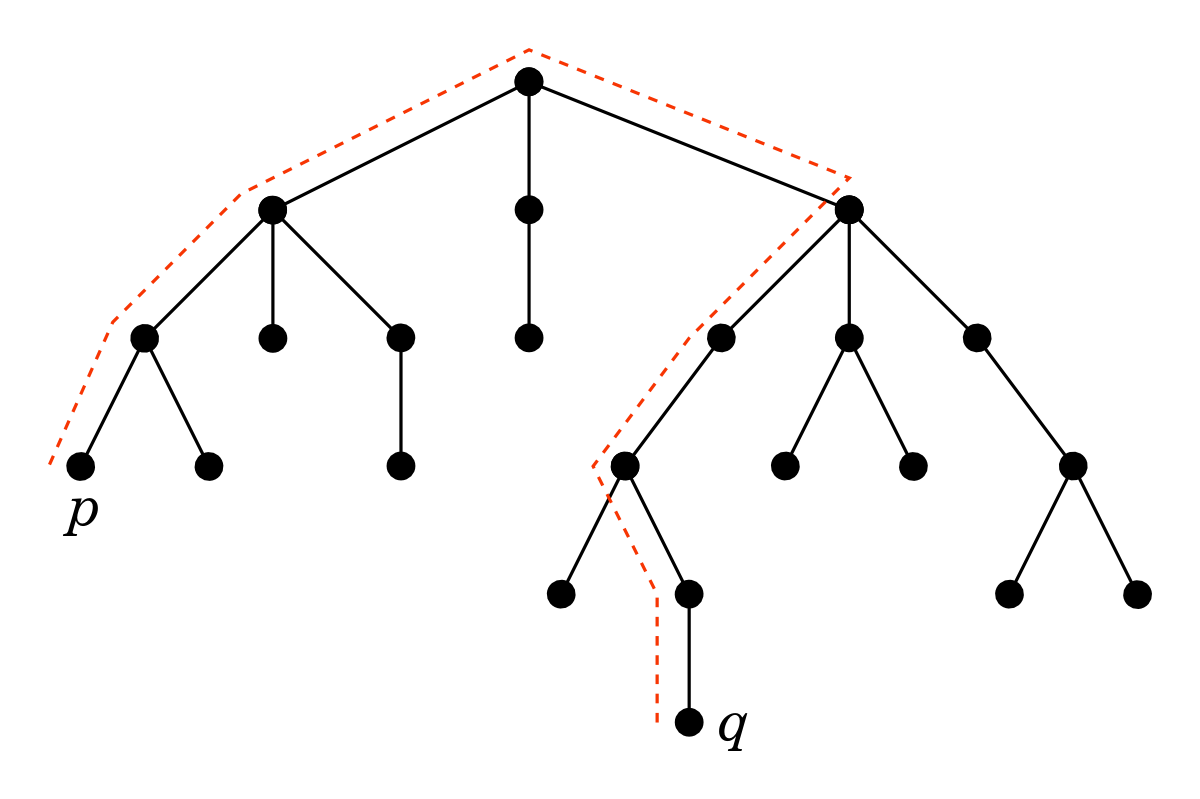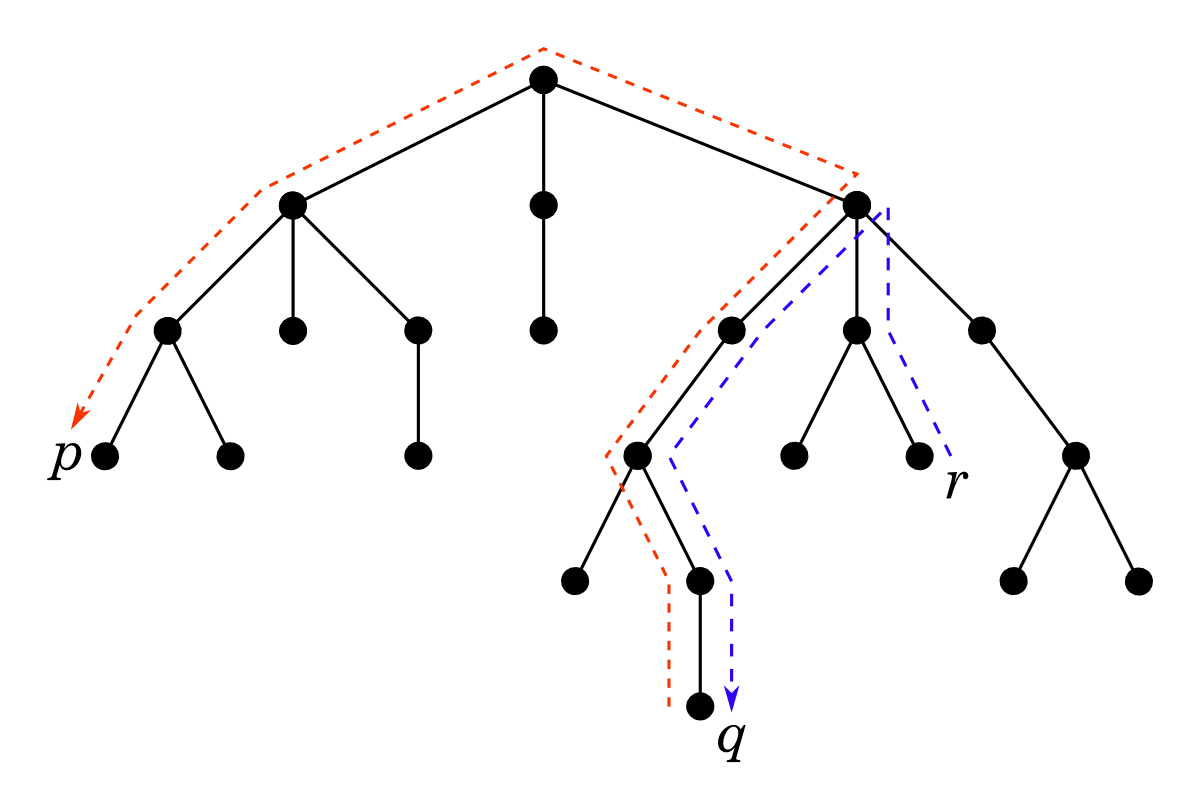# Walking on trees

Yesterday I learned a cool algorithm, which can be intuitively described as follows:

Pick a starting node, walk as far as you can, turn around, and walk as far as you can again.

Doesn’t sound too complicated, right? But it actually solves a non-trivial problem: it finds the diameter of a tree in linear computation time. The diameter of a tree is the length of the longest possible walk on it. For example, the tree below has diameter 8, because the longest walk on it goes through 8 links. There are actually three walks of length 8, we show the one between nodes p and q:How can we find the longest walk in a tree? A recursive algorithm comes to mind: solve the problem for subtrees of an arbitrary chosen root node and then combine the solutions somehow. Indeed this does work, but the implementation is not straightforward, in particular, one should carefully deal with two possible cases: i) the longest walk goes through the root (as in the above example), ii) the longest walk doesn’t go through the root. I recently tried to implement this during a programming contest (see this problem), but messed up and didn’t manage to debug my code in time.

There is a simpler algorithm, which is much easier to implement. Repeat after me:

Pick a starting node, walk as far as you can, turn around, and walk as far as you can again.

Let’s try it on our tree picking r as the starting node:The first ‘walk as far as you can’ step leads us to node q that is 6 links away (note that there are several other nodes that are as far as q, including node p, and we are free to choose any of them in this step). The second ‘walk as far as you can’ step leads us from q to p. That’s it: we found the diameter.

Why does this work? There are two key observations:

1. The first walk must stop at a node which is an endpoint of the tree’s diameter.
2. The second walk must stop at the other endpoint of the diameter.

The first observation is tricky and is a nice exercise to prove, which I leave to the reader, and the second one then follows directly. (You might want to have a look at this detailed correctness analysis.)

From the implementation point of view the algorithm is straightforward: simply pick your favourite algorithm for graph traversal, DFS or BFS, and use it for finding the farthest node from a given source node in a tree:

1. Pick s as the staring node.
2. Let u = farthest(s).
3. Let v = farthest(u).
4. Done: endpoints of the diameter are u and v.

One particularly interesting application for the algorithm is finding the shortest possible walk on the tree that visits each node at least once. A puzzle for the reader: try to see how knowing the diameter of the tree helps (this is not trivial). This was exactly the problem I was trying to solve at the programming contest I mentioned. Incidentally, this is a variant of the Travelling Salesman Problem that we looked into in the previous blog post.